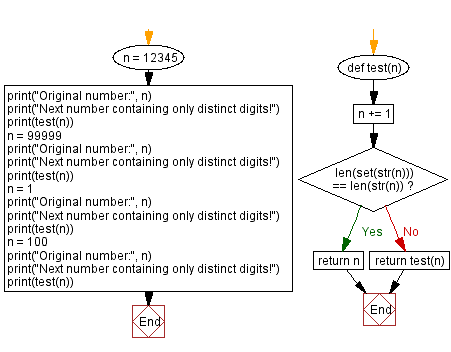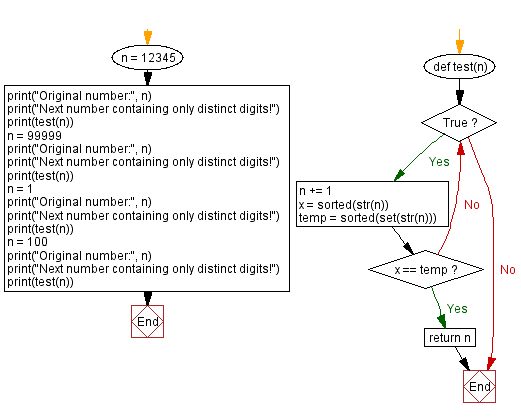﻿ Python - Next number containing only distinct digits

# Python Exercises: Next number containing only distinct digits

## Python Math: Exercise-91 with Solution

Write a Python program that accepts an integer number with distinct digits and displays the next number containing only distinct digits.

Sample Data:
12345) -> 12346
(99999) -> 102345
(100) -> 102

Sample Solution-1:

Python Code:

``````def test(n):
n += 1
if len(set(str(n))) == len(str(n)):
return n
return test(n)
n = 12345
print("Original number:", n)
print("Next number containing only distinct digits!")
print(test(n))
n = 99999
print("Original number:", n)
print("Next number containing only distinct digits!")
print(test(n))
n = 1
print("Original number:", n)
print("Next number containing only distinct digits!")
print(test(n))
n = 100
print("Original number:", n)
print("Next number containing only distinct digits!")
print(test(n))
```
```

Sample Output:

```Original number: 12345
Next number containing only distinct digits!
12346
Original number: 99999
Next number containing only distinct digits!
```

Flowchart:## Visualize Python code execution:

The following tool visualize what the computer is doing step-by-step as it executes the said program:

Sample Solution-2:

Python Code:

``````def test(n):
while True:
n += 1
x = sorted(str(n))
temp = sorted(set(str(n)))
if x == temp:
return n

n = 12345
print("Original number:", n)
print("Next number containing only distinct digits!")
print(test(n))
n = 99999
print("Original number:", n)
print("Next number containing only distinct digits!")
print(test(n))
n = 1
print("Original number:", n)
print("Next number containing only distinct digits!")
print(test(n))
n = 100
print("Original number:", n)
print("Next number containing only distinct digits!")
print(test(n))
```
```

Sample Output:

```Original number: 12345
Next number containing only distinct digits!
12346
Original number: 99999
Next number containing only distinct digits!
102345
Original number: 1
Next number containing only distinct digits!
2
Original number: 100
Next number containing only distinct digits!
102
```

Flowchart:## Visualize Python code execution:

The following tool visualize what the computer is doing step-by-step as it executes the said program:

Python Code Editor:

Have another way to solve this solution? Contribute your code (and comments) through Disqus.

Previous Python Exercise: Check if a number is a Harshad number or not.
Next Python Exercise: Absolute difference between two consecutive digits.

What is the difficulty level of this exercise?

Test your Programming skills with w3resource's quiz.

﻿

## Python: Tips of the Day

Creating a sequence of numbers (zero to ten with skips):

```>>> range(0,10,2)
[0, 2, 4, 6, 8]
```

We are closing our Disqus commenting system for some maintenanace issues. You may write to us at reach[at]yahoo[dot]com or visit us at Facebook